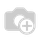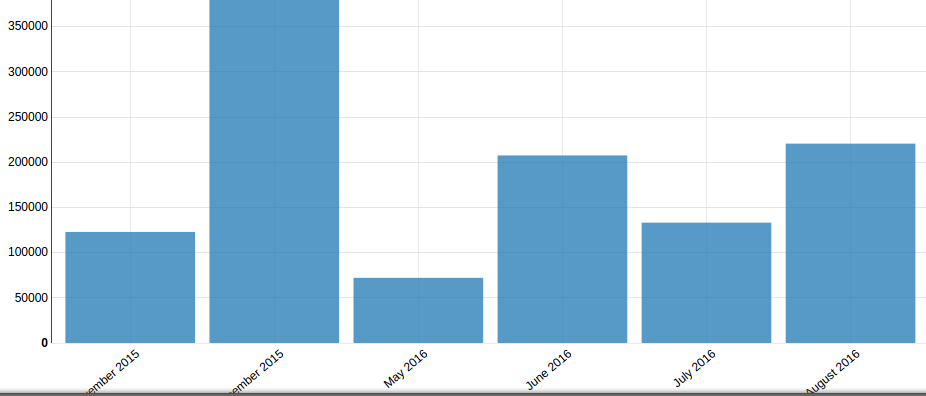# [ODOO 9] Graph Widget: How to customise the BAR Graph?

Hello,

I want to customise the GraphWidget: BAR Graph. In the graph_widget.js file there is the one option RotateLabels: 40, in chart.options({}) Dictionary. That option is commented. I want to print the labels rotate. When I remove tht comment it is working fine, But when I tried in my customise module, it is not working.

Please help me out for this. How to customize this JS file and how to work with it.Chandni

You just need to extend the proper widget class using include, like:

```var GraphWidget = require('web.GraphWidget');
GraphWidget.include({
display_bar: function () {
// prepare data for bar chart
var data, values,
measure = this.fields[this.measure].string;

// zero groupbys
if (this.groupbys.length === 0) {
data = [{
values: [{
x: measure,
y: this.data.value}],
key: measure
}];
}
// one groupby
if (this.groupbys.length === 1) {
values = this.data.map(function (datapt) {
return {x: datapt.labels, y: datapt.value};
});
data = [
{
values: values,
key: measure,
}
];
}
if (this.groupbys.length > 1) {
var xlabels = [],
series = [],
label, serie, value;
values = {};
for (var i = 0; i < this.data.length; i++) {
label = this.data[i].labels;
serie = this.data[i].labels;
value = this.data[i].value;
if ((!xlabels.length) || (xlabels[xlabels.length-1] !== label)) {
xlabels.push(label);
}
series.push(this.data[i].labels);
if (!(serie in values)) {values[serie] = {};}
values[serie][label] = this.data[i].value;
}
series = _.uniq(series);
data = [];
var current_serie, j;
for (i = 0; i < series.length; i++) {
current_serie = {values: [], key: series[i]};
for (j = 0; j < xlabels.length; j++) {
current_serie.values.push({
x: xlabels[j],
y: values[series[i]][xlabels[j]] || 0,
});
}
data.push(current_serie);
}
}
var svg = d3.select(this.\$el).append('svg');
svg.datum(data);

svg.transition().duration(0);

var chart = nv.models.multiBarChart();
var maxVal = _.max(values, function(v) {return v.y})
chart.options({
margin: {left: 12 * String(maxVal && maxVal.y || 0).length},
delay: 250,
transition: 10,
showLegend: _.size(data) <= MAX_LEGEND_LENGTH,
showXAxis: true,
showYAxis: true,
rightAlignYAxis: false,
stacked: this.stacked,
reduceXTicks: false,
rotateLabels: 40,
showControls: (this.groupbys.length > 1)
});
chart.yAxis.tickFormat(function(d) { return formats.format_value(d, { type : 'float' });});

chart(svg);
this.to_remove = chart.update;
nv.utils.onWindowResize(chart.update);
}
});```Hi Axel,

Thanks for your Quick response. I have already did this n it is resolved now. Thank u so much.

Do u know where is the code for the abbreviation of Months and Date everywhere in ODOO?

I am searching but could not find exact.

I tried this:

chart.xAxis.tickFormat(function (d) { return d3.time.format('%b')(new Date(d)); });

Just after the YAXIS, but it only returns the date everytime. It wont work with other values. ONly works When I filter group_by Months n Date.

Hi,

How did you get it to work?

I am trying Chandni's answer but when I put the code in my js file it fails to load other modules. I am on version 9.

`openerp.route_manager = function(instance, local) {    var _t = instance.web._t,        _lt = instance.web._lt;    var QWeb = instance.web.qweb;      var GraphWidget = require('web.GraphWidget');     GraphWidget.include({display_bar: function (){....}});};`
`What am I not getting or failing to doThe error I keep getting is "error: Some modules could not be started Failed modules:..." `Hi,

I want Abbreviation for this: Months and Year.